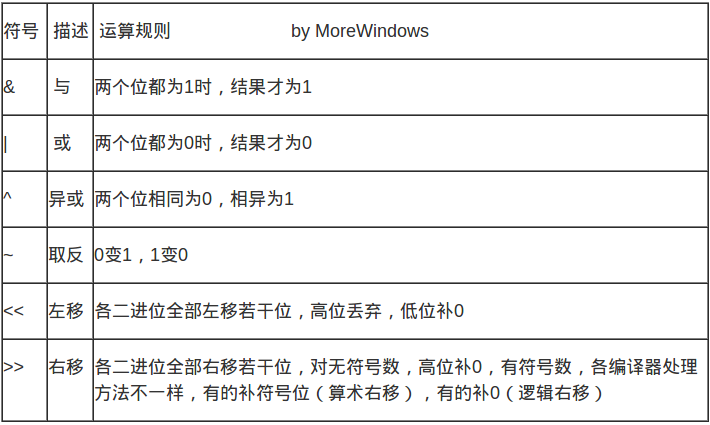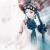## Java位操作全面总结 原

##位操作基础## 基本的位操作符有与、或、异或、取反、左移、右移这6种，它们的运算规则如下所示：注意以下几点：

1. 在这6种操作符，只有~取反是单目操作符，其它5种都是双目操作符。
2. 位操作只能用于整形数据，对float和double类型进行位操作会被编译器报错。
3. 位操作符的运算优先级比较低，因为尽量使用括号来确保运算顺序，否则很可能会得到莫明其妙的结果。比如要得到像1，3，5，9这些2^i+1的数字。写成int a = 1 << i + 1;是不对的，程序会先执行i + 1，再执行左移操作。应该写成int a = (1 << i) + 1;
4. 另外位操作还有一些复合操作符，如&=、|=、 ^=、<<=、>>=。
``````package com.king.bit;

/**
* @author taomk
* @version 1.0
* @since 15-5-10 下午2:23
*/
public class BitMain {

public static void main(String [] args) {
int a = -15, b = 15;
System.out.println(a >> 2); // -4：-15 = 1111 0001(二进制)，右移二位，最高位由符号位填充将得到1111 1100即-4
System.out.println(b >> 2); // 3：15=0000 1111(二进制)，右移二位，最高位由符号位填充将得到0000 0011即3
}
}
``````

##常用位操作小技巧## 下面对位操作的一些常见应用作个总结，有判断奇偶、交换两数、变换符号及求绝对值。这些小技巧应用易记，应当熟练掌握。 ###判断奇偶### 只要根据最未位是0还是1来决定，为0就是偶数，为1就是奇数。因此可以用if ((a & 1) == 0)代替if (a % 2 == 0)来判断a是不是偶数。下面程序将输出0到100之间的所有偶数：

``````    for (int i = 0; i < 100; i ++) {
if ((i & 1) == 0) { // 偶数
System.out.println(i);
}
}
``````

###交换两数###

``````int c = 1, d = 2;
c ^= d;
d ^= c;
c ^= d;
System.out.println("c=" + c);
System.out.println("d=" + d);
``````

1111 0101(二进制) –取反-> 0000 1010(二进制) –加1-> 0000 1011(二进制)

0000 1011(二进制) –取反-> 0000 0100(二进制) –加1-> 1111 0101(二进制)

``````int a = -15, b = 15;
System.out.println(~a + 1);
System.out.println(~b + 1);
``````

###求绝对值### 位操作也可以用来求绝对值，对于负数可以通过对其取反后加1来得到正数。对-6可以这样：

1111 1010(二进制) –取反->0000 0101(二进制) -加1-> 0000 0110(二进制)

``````int i = a >> 31;
System.out.println(i == 0 ? a : (~a + 1));
``````

``````int j = a >> 31;
System.out.println((a ^ j) - j);
``````

``````// 打印100以内素数：
// （1）对每个素数，它的倍数必定不是素数；
// （2）有很多重复访问如flag会在访问flag和flag时各访问一次；
int max = 100;
boolean[] flags = new boolean[max];
int [] primes = new int[max / 3 + 1];
int pi = 0;

for (int m = 2; m < max ; m ++) {
if (!flags[m]) {
primes[pi++] = m;
for(int n = m; n < max; n += m) {
flags[n] = true;
}
}
}

System.out.println(Arrays.toString(primes));
``````

[2, 3, 5, 7, 11, 13, 17, 19, 23, 29, 31, 37, 41, 43, 47, 53, 59, 61, 67, 71, 73, 79, 83, 89, 97, 0, 0, 0, 0, 0, 0, 0, 0, 0]

``````// 在一个数指定位上置1
int e = 0;
e |=  1 << 10;
System.out.println(e);
``````

``````//判断指定位上是0还是1
if ((e & (1 << 10)) != 0)
System.out.println("指定位上为1");
else
System.out.println("指定位上为0");
``````

``````int[] bits = new int;
for (int m = 0; m < 40; m += 3) {
bits[m / 32] |= (1 << (m % 32));
}
// 输出整个bits
for (int m = 0; m < 40; m++) {
if (((bits[m / 32] >> (m % 32)) & 1) != 0)
System.out.print('1');
else
System.out.print('0');
}
``````

1001001001001001001001001001001001001001

``````int[] flags2 = new int[max / 32 + 1];
pi = 0;
for (int m = 2; m < max ; m ++) {
if ((((flags2[m / 32] >> (m % 32)) & 1) == 0)) {
primes[pi++] = m;
for(int n = m; n < max; n += m) {
flags2[n / 32] |= (1 << (n % 32));
}
}
}

System.out.println();
System.out.println(Arrays.toString(primes));
``````

[2, 3, 5, 7, 11, 13, 17, 19, 23, 29, 31, 37, 41, 43, 47, 53, 59, 61, 67, 71, 73, 79, 83, 89, 97, 0, 0, 0, 0, 0, 0, 0, 0, 0]

##位操作工具类##

``````package com.king.bit;

/**
* Java 位运算的常用方法封装<br>
*/
public class BitUtils {

/**
* 获取运算数指定位置的值<br>
* 例如： 0000 1011 获取其第 0 位的值为 1, 第 2 位 的值为 0<br>
*
* @param source
*            需要运算的数
* @param pos
*            指定位置 (0<=pos<=7)
* @return 指定位置的值(0 or 1)
*/
public static byte getBitValue(byte source, int pos) {
return (byte) ((source >> pos) & 1);
}

/**
* 将运算数指定位置的值置为指定值<br>
* 例: 0000 1011 需要更新为 0000 1111, 即第 2 位的值需要置为 1<br>
*
* @param source
*            需要运算的数
* @param pos
*            指定位置 (0<=pos<=7)
* @param value
*            只能取值为 0, 或 1, 所有大于0的值作为1处理, 所有小于0的值作为0处理
*
* @return 运算后的结果数
*/
public static byte setBitValue(byte source, int pos, byte value) {

byte mask = (byte) (1 << pos);
if (value > 0) {
} else {
}

return source;
}

/**
* 将运算数指定位置取反值<br>
* 例： 0000 1011 指定第 3 位取反, 结果为 0000 0011; 指定第2位取反, 结果为 0000 1111<br>
*
* @param source
*
* @param pos
*            指定位置 (0<=pos<=7)
*
* @return 运算后的结果数
*/
public static byte reverseBitValue(byte source, int pos) {
byte mask = (byte) (1 << pos);
return (byte) (source ^ mask);
}

/**
* 检查运算数的指定位置是否为1<br>
*
* @param source
*            需要运算的数
* @param pos
*            指定位置 (0<=pos<=7)
* @return true 表示指定位置值为1, false 表示指定位置值为 0
*/
public static boolean checkBitValue(byte source, int pos) {

source = (byte) (source >>> pos);

return (source & 1) == 1;
}

/**
* 入口函数做测试<br>
*
* @param args
*/
public static void main(String[] args) {

// 取十进制 11 (二级制 0000 1011) 为例子
byte source = 11;

// 取第2位值并输出, 结果应为 0000 1011
for (byte i = 7; i >= 0; i--) {
System.out.printf("%d ", getBitValue(source, i));
}

// 将第6位置为1并输出 , 结果为 75 (0100 1011)
System.out.println("\n" + setBitValue(source, 6, (byte) 1));

// 将第6位取反并输出, 结果应为75(0100 1011)
System.out.println(reverseBitValue(source, 6));

// 检查第6位是否为1，结果应为false
System.out.println(checkBitValue(source, 6));

// 输出为1的位, 结果应为 0 1 3
for (byte i = 0; i < 8; i++) {
if (checkBitValue(source, i)) {
System.out.printf("%d ", i);
}
}

}
}
``````

##BitSet类## BitSet类：大小可动态改变, 取值为true或false的位集合。用于表示一组布尔标志。 此类实现了一个按需增长的位向量。位 set 的每个组件都有一个 boolean 值。用非负的整数将 BitSet 的位编入索引。可以对每个编入索引的位进行测试、设置或者清除。通过逻辑与、逻辑或和逻辑异或操作，可以使用一个 BitSet 修改另一个 BitSet 的内容。默认情况下，set 中所有位的初始值都是 false。

public void set(int pos): 位置pos的字位设置为true。 public void set(int bitIndex, boolean value): 将指定索引处的位设置为指定的值。 public void clear(int pos): 位置pos的字位设置为false。 public void clear(): 将此 BitSet 中的所有位设置为 false。 public int cardinality(): 返回此 BitSet 中设置为 true 的位数。 public boolean get(int pos): 返回位置是pos的字位值。 public void and(BitSet other): other同该字位集进行与操作，结果作为该字位集的新值。 public void or(BitSet other): other同该字位集进行或操作，结果作为该字位集的新值。 public void xor(BitSet other): other同该字位集进行异或操作，结果作为该字位集的新值。 public void andNot(BitSet set): 清除此 BitSet 中所有的位,set - 用来屏蔽此 BitSet 的 BitSet public int size(): 返回此 BitSet 表示位值时实际使用空间的位数。 public int length(): 返回此 BitSet 的“逻辑大小”：BitSet 中最高设置位的索引加 1。 public int hashCode(): 返回该集合Hash 码， 这个码同集合中的字位值有关。 public boolean equals(Object other): 如果other中的字位同集合中的字位相同，返回true。 public Object clone(): 克隆此 BitSet，生成一个与之相等的新 BitSet。 public String toString(): 返回此位 set 的字符串表示形式。

``````package com.king.bit;

import java.util.BitSet;

public class WhichChars {

private BitSet used = new BitSet();

public WhichChars(String str) {
for (int i = 0; i < str.length(); i++)
used.set(str.charAt(i));  // set bit for char
}

public String toString() {
String desc = "[";
int size = used.size();
for (int i = 0; i < size; i++) {
if (used.get(i))
desc += (char) i;
}
return desc + "]";
}

public static void main(String args[]) {
WhichChars w = new WhichChars("How do you do");
System.out.println(w);
}
}
``````

``````package com.king.bit;

import java.util.BitSet;

public class MainTestThree {

/**
* @param args
*/
public static void main(String[] args) {
BitSet bm = new BitSet();
System.out.println(bm.isEmpty() + "--" + bm.size());
bm.set(0);
System.out.println(bm.isEmpty() + "--" + bm.size());
bm.set(1);
System.out.println(bm.isEmpty() + "--" + bm.size());
System.out.println(bm.get(65));
System.out.println(bm.isEmpty() + "--" + bm.size());
bm.set(65);
System.out.println(bm.isEmpty() + "--" + bm.size());
}

}
``````

``````package com.king.bit;

import java.util.BitSet;

public class MainTestFour {

/**
* @param args
*/
public static void main(String[] args) {
BitSet bm1 = new BitSet(7);
System.out.println(bm1.isEmpty() + "--" + bm1.size());

BitSet bm2 = new BitSet(63);
System.out.println(bm2.isEmpty() + "--" + bm2.size());

BitSet bm3 = new BitSet(65);
System.out.println(bm3.isEmpty() + "--" + bm3.size());

BitSet bm4 = new BitSet(111);
System.out.println(bm4.isEmpty() + "--" + bm4.size());
}

}
``````

##位操作技巧##

``````// 1. 获得int型最大值
System.out.println((1 << 31) - 1);// 2147483647， 由于优先级关系，括号不可省略
System.out.println(~(1 << 31));// 2147483647

// 2. 获得int型最小值
System.out.println(1 << 31);
System.out.println(1 << -1);

// 3. 获得long类型的最大值
System.out.println(((long)1 << 127) - 1);

// 4. 乘以2运算
System.out.println(10<<1);

// 5. 除以2运算(负奇数的运算不可用)
System.out.println(10>>1);

// 6. 乘以2的m次方
System.out.println(10<<2);

// 7. 除以2的m次方
System.out.println(16>>2);

// 8. 判断一个数的奇偶性
System.out.println((10 & 1) == 1);
System.out.println((9 & 1) == 1);

// 9. 不用临时变量交换两个数（面试常考）
a ^= b;
b ^= a;
a ^= b;

// 10. 取绝对值（某些机器上，效率比n>0 ? n:-n 高）
int n = -1;
System.out.println((n ^ (n >> 31)) - (n >> 31));
/* n>>31 取得n的符号，若n为正数，n>>31等于0，若n为负数，n>>31等于-1

// 11. 取两个数的最大值（某些机器上，效率比a>b ? a:b高）
System.out.println(b&((a-b)>>31) | a&(~(a-b)>>31));

// 12. 取两个数的最小值（某些机器上，效率比a>b ? b:a高）
System.out.println(a&((a-b)>>31) | b&(~(a-b)>>31));

// 13. 判断符号是否相同(true 表示 x和y有相同的符号， false表示x，y有相反的符号。)
System.out.println((a ^ b) > 0);

// 14. 计算2的n次方 n > 0
System.out.println(2<<(n-1));

// 15. 判断一个数n是不是2的幂
System.out.println((n & (n - 1)) == 0);
/*如果是2的幂，n一定是100... n-1就是1111....

// 16. 求两个整数的平均值
System.out.println((a+b) >> 1);

// 17. 从低位到高位,取n的第m位
int m = 2;
System.out.println((n >> (m-1)) & 1);

// 18. 从低位到高位.将n的第m位置为1
System.out.println(n | (1<<(m-1)));
/*将1左移m-1位找到第m位，得到000...1...000
n在和这个数做或运算*/

// 19. 从低位到高位,将n的第m位置为0
System.out.println(n & ~(0<<(m-1)));
/* 将1左移m-1位找到第m位，取反后变成111...0...1111
n再和这个数做与运算*/
``````### 评论(1)Java I/O : Java中的进制详解

2015/06/14
449
0
【有奖书评】JAVA 7来袭，还看 Java 你就OUT了

louise
2012/08/31
12.5K
123
java 时间戳和PHP时间戳 的转换 php time()

2013/08/04
2.4K
0
Java开发基础(二)——JDBC的使用

simon曦
05/22
0
0

Java-飞鱼
09/11
60
0

html2canvas 生成的图片变模糊解决方案

25分钟前
6
0

401恶户
26分钟前
6
0

31分钟前
7
0

9月4日，第十届中国电子商务物流大会暨2019中国同城即时物流行业峰会于杭州召开。会上出现了蜂鸟即配、达达-京东到家、美团配送、点我达、闪送等一众即时配送行业中的知名企业的身影。 在艾媒...

31分钟前
6
0
Moto G 2014 LTE Root

[TOC] 下载moto专用mfastboot mfastboot-v2.zip 下载对应设备的twrp 我的是这个版本 Moto G 2014 LTE thea 刷入twrp # 1. 开启USE调试# 2. 允许OEM解锁# 3. 查看设备adb devices# 4. 进f...

yeahlife
35分钟前
5
0